Related Articles
Pass by reference vs value in Python
• Last Updated : 10 May, 2020

Developers jumping into Python programming from other languages like C++ and Java are often confused by the process of passing arguments in Python. The object-centric data model and its treatment of assignment is the cause behind the confusion at the fundamental level. In the article below we will be discussing the concept of passing arguments in Python and try to understand it with the help of examples.

### Is Python Argument Passing model a “Pass by Value” or “Pass by Reference”?

You might want to punch something after reading ahead, so brace your self. Python’s argument passing model is neither “Pass by Value” nor “Pass by Reference” but it is “Pass by Object Reference”.

The paradigms of “Pass by value”, “Pass by Reference” and “Pass by object Reference” can be understood by exploring the below example functions.
Look into the two functions defined below:

 `def` `set_list(``list``):``    ``list` `=` `[``"A"``, ``"B"``, ``"C"``]``    ``return` `list`` ` `def` `add(``list``):``    ``list``.append(``"D"``)``    ``return` `list`` ` `my_list ``=` `[``"E"``]`` ` `print``(set_list(my_list))`` ` `print``(add(my_list))`

Output:

```['A', 'B', 'C']
['E', 'D']```

Now, let’s explore the above code,

The variable is not the object passed:
What if I tell you The Ramayana was not written by Tulsi Das but The Ramayana was written by a man named Tulsi Das. Does that distinction make any sense? No right?!. But according to Python it does and it makes a crucial distinction. So, in Python and its PKD’s there is a big difference between the thing and the label we use to refer to that thing. “A man named Tulsi Das ” is just a man and “Tulsi Das” is a name used to refer to that man.
So, consider a list

` a = ["X", "Y"]`

Here “a” is a variable that points to a list containing the element “X” and “Y”. But “a” itself is not the list. Consider “a” to be a bucket that contains the object “X” and “Y”.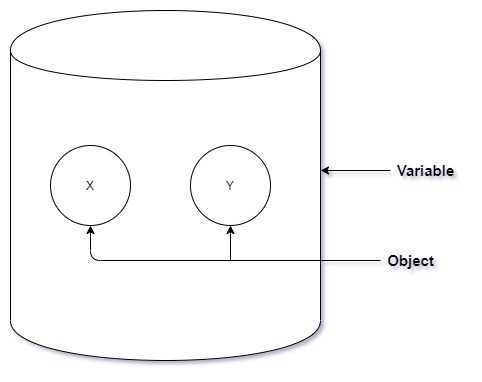Pass by Reference:

In pass by reference the variable( the bucket) is passed into the function directly. The variable acts a Package that comes with it’s contents(the objects).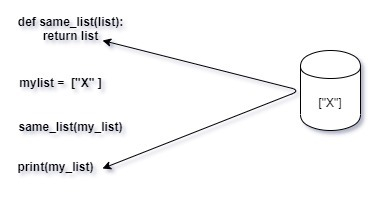In the above code image both “list” and “my_list” are the same container variable and therefore refer to the exact same object in the memory. Any operation performed by the function on the variable or the object will be directly reflected to the function caller. For instance, the function could completely change the variable’s content, and point it at a completely different object: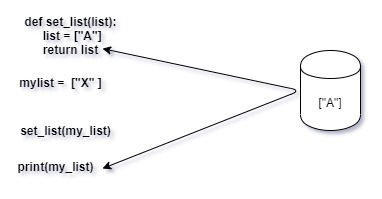Also, the function can reassign the contents of the variable with the same effect as below: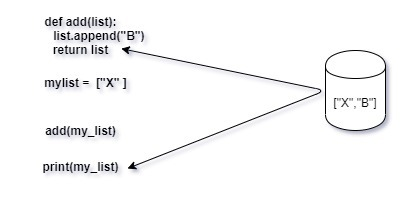To summarise, in pass by reference the function and the caller use the same variable and object.

Pass by Value:

In pass by value the function is provided with a copy of the argument object passed to it by the caller. That means the original object stays intact and all changes made are to a copy of the same and stored at different memory locations.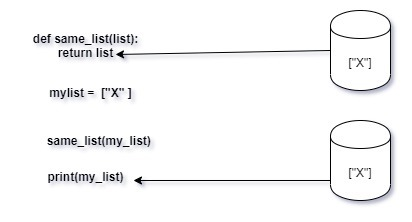The same is true for any operation performed by the function on the variable or the object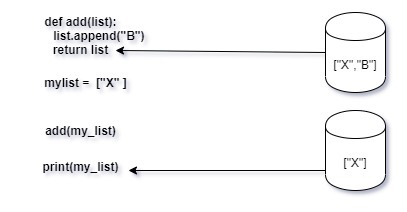To summarise the copies of the variables and the objects in the context of the caller of the function are completely isolated.

Pass Object by Reference:

As python is different in this context, the functions in python receive the reference of the same object in the memory as referred by the caller. However, the function does not receive the variable(the bucket) that the caller is storing the same object in; like in pass-by-value, the function provides its own bucket and creates an entirely new variable for itself.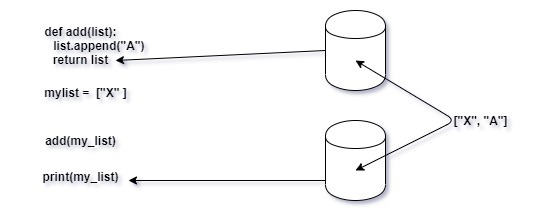The same object in the memory is referred by both the caller and the function, so when the append function adds an extra element to the list, the caller object gets updated too. They have different names but are the same thing. Both the variables contain the same object. This is the meaning behind pass by object reference. The function and caller use the same object in memory, but get them through different variables. Any changes made to the function variable(bucket) won’t change the nature of the caller variable (bucket), only the content gets updated.

Attention geek! Strengthen your foundations with the Python Programming Foundation Course and learn the basics.

To begin with, your interview preparations Enhance your Data Structures concepts with the Python DS Course. And to begin with your Machine Learning Journey, join the Machine Learning – Basic Level Course

My Personal Notes arrow_drop_up